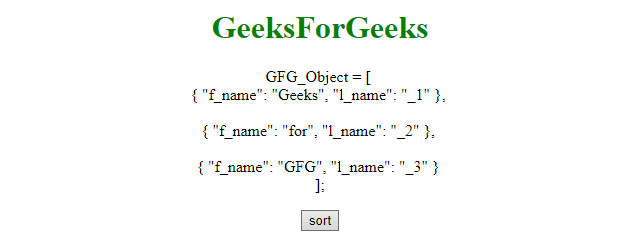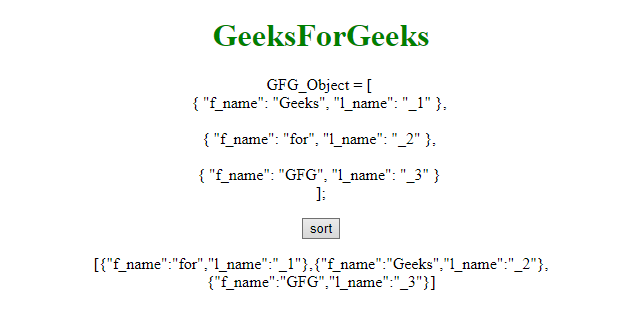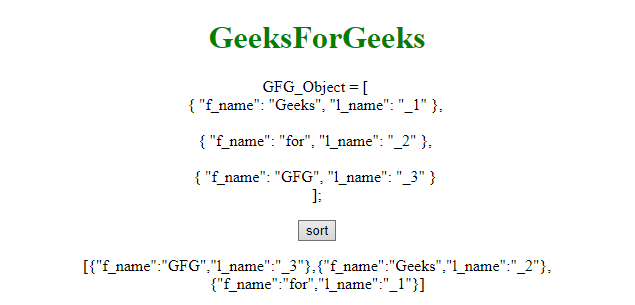Related Articles

# Sort array of objects by string property value in JavaScript

• Last Updated : 28 Mar, 2019

The array of objects can be sort by using user defined function. This function compares the array of objects by its property. For example, the first example compares the l_name of objects and if l_name is small then it place into left otherwise place it into right position.

Example 1: This example sorts the array of objects by l_name property.

 ` ``<``html``> `` ` `<``head``> ``    ``<``title``> ``        ``Sort array of objects``    ```` ``         ` `<``body` `style` `= ``"text-align:center;"``> ``     ` `    ``<``h1` `style` `= ``"color:green;"` `> ``        ``GeeksForGeeks ``    `` ``     ` `    ``<``p` `id``=``"demo2"``>GFG_Object = [ <``br``>``        ``{ "f_name": "Geeks", "l_name": "_1" },``        ``<``br``><``br``>``        ``{ "f_name": "for", "l_name": "_2" },``        ``<``br``><``br``>``        ``{ "f_name": "GFG", "l_name": "_3" }``        ``<``br``>];``    `` ``     ` `    ``<``button` `onClick``=``"fun()"``>``        ``sort``    ````     ` `    ``<``p` `id``=``"GFG"``> ``     ` `    ````    ``<``script``>``        ``function fun() {``            ``function compare(a, b) {``                ``if (a.l_name < ``b.l_name``)``                    ``return -1;``                ``if (a.l_name > b.l_name)``                    ``return 1;``                ``return 0;``            ``}``            ``var GFG_Object = [ ``                ``{ f_name: 'Geeks', l_name: '_2' },``                ``{ f_name: 'for', l_name: '_1' },``                ``{ f_name: 'GFG', l_name: '_3' }``            ``];``             ` `            ``GFG_Object.sort(compare);``             ` `            ``document.getElementById("GFG").innerHTML``                    ``= JSON.stringify(GFG_Object);``        ``}``        `` ``    `` ``                    `

Output:

• Before click on the button:• After click on the button:Example 2: This example sorts the array of objects by f_name property.

 ` ``<``html``> `` ` `<``head``> ``    ``<``title``> ``        ``Sort array of objects``    ```` ``         ` `<``body` `style` `= ``"text-align:center;"``> ``     ` `    ``<``h1` `style` `= ``"color:green;"` `> ``        ``GeeksForGeeks ``    `` ``     ` `    ``<``p` `id``=``"demo2"``> GFG_Object = [ <``br``>``        ``{ "f_name": "Geeks", "l_name": "_1" },``        ``<``br``><``br``>``        ``{ "f_name": "for", "l_name": "_2" },``        ``<``br``><``br``>``        ``{ "f_name": "GFG", "l_name": "_3" }``        ``<``br``>];``    `` ``     ` `    ``<``button` `onClick``=``"fun()"``>``        ``sort``    ````     ` `    ``<``p` `id``=``"GFG"``> `` ` `    ``<``script``>``        ``function fun() {``            ``function compare(a, b) {``                ``if (a.f_name < ``b.f_name``)``                    ``return -1;``                ``if (a.f_name > b.f_name)``                    ``return 1;``                ``return 0;``            ``}``            ``var GFG_Object = [ ``                ``{ f_name: 'Geeks', l_name: '_2' },``                ``{ f_name: 'for', l_name: '_1' },``                ``{ f_name: 'GFG', l_name: '_3' }``            ``];``             ` `            ``GFG_Object.sort(compare);``             ` `            ``document.getElementById("GFG").innerHTML``                    ``= JSON.stringify(GFG_Object);``        ``}``    `` `` `` ` `                    `

Output:

• Before click on the button:• After click on the button:Hey geek! The constant emerging technologies in the world of web development always keeps the excitement for this subject through the roof. But before you tackle the big projects, we suggest you start by learning the basics. Kickstart your web development journey by learning JS concepts with our JavaScript Course. Now at it’s lowest price ever!

My Personal Notes arrow_drop_up